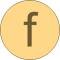# Helmholtz_ph

Function to calculate analytic derivatives for computing d and t given p and h# Syntax

nderivs = Helmholtz_ph(f)

# Inputs (1)

f Type: HelmholtzDerivs Description: Dimensionless derivatives of Helmholtz function

# Outputs (1)

nderivs Type: NewtonDerivatives_ph Description: Derivatives for Newton iteration to calculate d and t from p and h